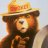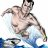# Moving average slope problem

M

#### msammons

##### New member
The code below (1) calculates the moving average slope, and then (2) should give the price difference between the moving average and the current stock price DEPENDING UPON THE SLOPE - but the "if" part is wrong - can anyone see the error?

#slope

def EMA30 = MovAvgExponential(close,30);
def slopeavg = Average(close,3);
def height = slopeavg - slopeavg;
def slope = Atan(height/3)*180/Double.Pi;

#conditions

def Condition1 = (slope < 0 and close > EMA30);
def Conditiion2 = (slope > 0 and close < EMA30);

#watchlist column result

plot X = if Condition1 then close - EMA30:
plot X = if Condition2 then EMA30 - close;#### horserider

##### Well-known member
VIP
As a reserved word, if is used in if-expressions and if-statements to specify a conditional operator with then and elsebranches. Both branches are required for the operator to be valid. However, while the if-expression always calculates both then and else branches, the if-statement only calculates the branch defined by whether the condition is true or false. In thinkScript®, there is also If-function having syntax and usage different from those of the reserved word. The if-expression can also be used in other functions such as, for example, AssignValueColor, AssignPriceColor, etc. Note that you can also use a def variable instead of myHigh. If the high price is greater, the value of myHigh is rewritten, otherwise the variable keeps its previous value as defined by the else branch.

•markos#### horserider

##### Well-known member
VIP
or

Returns true value if condition is true and false value otherwise. There are two ways to use the function. First, you can use it as the right side of an equation with 3 parameters: a condition, a true value and a false value. Secondly, you can use it in a conjunction with else to create more complex conditions.

Note that input arguments can only be numerical of type double. If other values are needed (e.g. Color constants), use the if-expression. E.g. for CustomColor arguments, the following code is valid:

AssignPriceColor(if close > open then Color.UPTICK else Color.DOWNTICK);
The following script will result in compilation error as type CustomColor is not compatible with type double:

AssignPriceColor(if(close > open, Color.UPTICK, Color.DOWNTICK));

Input parameters

ParameterDefault valueDescription
condition-Defines condition to be tested.
true value-Defines value to be returned if condition is true.
false value-Defines value to be returned if condition is false.

Example

plot Maximum1 = If(close > open, close, open);
plot Maximum2 = if close > open then close else open;
plot Maximum3;
if close > open {
Maximum3 = close;
} else {
Maximum3 = open;
}

The code draws either close or open value depending on the condition in the if-statement. If close is higher than open, then close is drawn, otherwise open is drawn. Maximum2 and Maximum3 plots use alternative solutions such as if-expression and if-statement correspondingly.

M

#### msammons

##### New member
horserider: thank you for copying/pasting all that from the TOS thinkscript center - however it is still not clear to me where my error is (and I am not sure how all that about colors, type doubles, assigncolor up-down ticks, etc you posted applies to my question).

I "think" my slope part is correct.
I "think" my conditions part is correct.

I "know" the Plot X part is wrong.

If someone agrees that my slope and conditions parts are correct (I get no error codes), I would really appreciate a suggestion on how to correct the plot part.

M

#### msammons

##### New member
I believe this might work (used excel nesting if statement idea):

#slope

def EMA30 = MovAvgExponential(close,30);
def slopeavg = Average(close,3);
def height = slopeavg - slopeavg;
def slope = Atan(height/3)*180/Double.Pi;

#conditions

def Condition1 = (slope < 0 and close > EMA30);
def Condition2 = (slope > 0 and close < EMA30);

#watchlist column result

plot x = if(Condition2,EMA30-close,if(Condition1,close-EMA30,0));#### horserider

##### Well-known member
VIP
Maybe ? ??

plot x = if Condition1 then close - EMA30 else if Condition2 then EMA30 - close else double.NaN;

M

#### msammons

##### New member
Thank you horserider - exactly what I was looking for. I will try out tomorrow when I have live data (TOS on-demand seems to have parts with delay).

T

#### tomsk

##### Well-known member
VIP
@msammons I looked into your query and ran a few tests. Looks like condition 1 may never be triggered in which case you may have to adjust your definition of condition 1. Before you plot anything on a watchlist, always verify your output on a chart. Use the following code snippet. If iyou are satisfied this is the output you want then you can implement this on a watchlist

No sense in waiting till tomorrow when you can verify your code conditions right now against a chart. Use a lower aggregation for clearer results

Code:
``````declare lower;

input length = 3;
def EMA30 = MovAvgExponential(close,length);
def slopeavg = Average(close,length);
def height = slopeavg - slopeavg[length];
def slope = Atan(height/length)*180/Double.Pi;

def Condition1 = (slope < 0 and close > EMA30);
def Condition2 = (slope > 0 and close < EMA30);

plot x = if Condition2 then EMA30-close else if Condition1 then close-EMA30 else 0;``````

Last edited:
M

#### msammons

##### New member
tomsk -thank you do much for your help!

I am confused why condition1 can never produce results. On a chart I am using an EMA30 (exponential moving average of 30 bars) to determine (a) EMA30 value for the last close, and (b) to eyeball slope. I am only interested in the difference between close and EMA30 if (a) the close is below an upsloping EMA30 slope line, or (b) the close is above a down-sloping EMA30 trend line. In those two situations I just want the difference in the close and EMA30 values.

Your code seems to set the length for both the slope calculation and the EMA at the same 3 bars (while I use 3 bars for slope and 30 bars for the EMA)- why did you use an EMA3 value rather than an EMA30 value?

But you code results in value of a few cents (which is correct for SMA30 - close), while when I use length 3 for slope and length 30 for SMA30 I get ridiculously large differences (over \$1 rather than the correct few cents).

Where am I off base?

M

#### msammons

##### New member
tomsk - does this look right (just uses EMA30 - EMA30 with displace 1, to determine slope):

def EMA30 = MovAvgExponential("length" = 30);

def Condition1 = MovAvgExponential("length" = 30)."AvgExp" is greater than MovAvgExponential("length" = 30, "displace" = 1)."AvgExp" and close is greater than MovAvgExponential("length" = 30)."AvgExp";

def Condition2 = MovAvgExponential("length" = 30)."AvgExp" is less than MovAvgExponential("length" = 30, "displace" = 1)."AvgExp" and close is less than MovAvgExponential("length" = 30)."AvgExp";

plot x = if Condition2 then EMA30-close else if Condition1 then close-EMA30 else 0;

Last edited:
T

#### tomsk

##### Well-known member
VIP
Before you write any more code, suggest you write a paragraph in english which describes what precisely you're looking for including the conditions. Writing the code is the easy part, it must be based on a clear description. This will help any scripters out there to better assist you

S

#### srinpraveen

##### New member
Hi all,
I am trying to build a partial version of the popular Mark Minervini template for scanning stocks using ThinkScript. There is 1 condition that I am stuck on at the moment. Is there a way in thinkscript to find if a particular Simple Moving Average is trending up for the last 30 days? In other words, I need to find out if the moving average is maintaining a positive slope in the past 30 days.

Thanks in advance for any help rendered on this topic.#### Prison Mike

##### Member
Maybe a simple way to do it would be set the time agg “month” and using something like month >month

S

#### srinpraveen

##### New member
Prison Mike, would this do what I think it would?
Code:
``DailySMA("length" = 200) is greater than DailySMA("length" = 200) from 30 bars ago``

I tried changing the aggregate period to month in this study function parameter but I got the error "secondary period not allowed:month". This might be because I set my length of the SMA to 200 days and the aggregation period to a month. This perhaps may have caused the function to bomb out. Not sure.#### Prison Mike

##### Member
@srinpraveen yea can’t have two time aggs in a scan. Trying to give you a simple solution. I know enough to get by but really need to be at my computer to mess around with it.
Here’s another idea. Keep it daily but use two lengths. One 199 the other 200.

But also my first suggestion should be easy using “condition wizard”

S

#### srinpraveen

##### New member
@Prison Mike , I used the condition wizard in the custom filter for scan to construct the code attached previously. DailySMA was the study name. Essentially, my offset value was "30" which represents last 30 days. This translates out to "from 30 bars ago" in thinkScript syntax. The length of the SMA computation was 200 days. Aggregate-period was set to "daily". This seems to work out well. I checked out the scan results on NASDAQ 100 stocks and compared it with the 200 day SMA chart-plots for a few scan results to make sure. The script seems to be doing what it was meant to do.Midpoint Moving Average Indicator Questions 2Moving average cloud? Questions 6ATR Trailing Stop (5atr period.1.5 factor) crossing 50period moving average with Alarm Questions 0